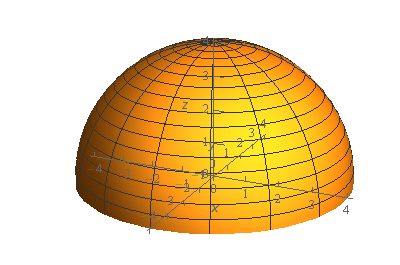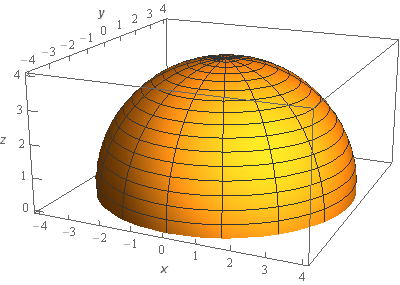Paul's Online Notes
Home / Calculus III / Multiple Integrals / Triple Integrals in Spherical Coordinates
Show Mobile Notice Show All Notes Hide All Notes
Mobile Notice
You appear to be on a device with a "narrow" screen width (i.e. you are probably on a mobile phone). Due to the nature of the mathematics on this site it is best views in landscape mode. If your device is not in landscape mode many of the equations will run off the side of your device (should be able to scroll to see them) and some of the menu items will be cut off due to the narrow screen width.

### Section 15.7 : Triple Integrals in Spherical Coordinates

1. Evaluate $$\displaystyle \iiint\limits_{E}{{10xz + 3\,dV}}$$ where $$E$$ is the region portion of $${x^2} + {y^2} + {z^2} = 16$$ with $$z \ge 0$$.

Show All Steps Hide All Steps

Start Solution

Okay, let’s start off with a quick sketch of the region $$E$$ so we can get a feel for what we’re dealing with.We’ve given the sketches with a set of “traditional” axes as well as a set of “box” axes to help visualize the surface and region.

In this case we’re dealing with the upper half of a sphere of radius 4.

Show Step 2

Now, since we are integrating over a portion of a sphere it makes sense to use spherical coordinate for the integral and the limits are,

$\begin{array}{c}0 \le \varphi \le \frac{\pi }{2}\\ 0 \le \theta \le 2\pi \\ 0 \le \rho \le 4\end{array}$

Remember that $$\varphi$$ is the angle from the positive $$z$$-axis that we rotate through as we cover the region and $$\theta$$ is the angle we rotate around the $$z$$-axis as we cover the region.

In this case we have the full upper half of the sphere and so $$\theta$$ will range from 0 to $$2\pi$$ while $$\varphi$$ will range from 0 to $$\frac{\pi }{2}$$.

Show Step 3

Plugging these limits into the integral and converting to spherical coordinates gives,

\begin{align*}\iiint\limits_{E}{{10xz + 3\,dV}}& = \int_{0}^{{\frac{\pi }{2}}}{{\int_{0}^{{2\pi }}{{\int_{0}^{4}{{\left[ {10\left( {\rho \sin \varphi \cos \theta } \right)\left( {\rho \cos \varphi } \right) + 3} \right]\left( {{\rho ^2}\sin \varphi } \right)\,d\rho }}\,d\theta }}\,d\varphi }}\\ & = \int_{0}^{{\frac{\pi }{2}}}{{\int_{0}^{{2\pi }}{{\int_{0}^{4}{{10{\rho ^4}{{\sin }^2}\varphi \cos \varphi \cos \theta + 3{\rho ^2}\sin \varphi \,d\rho }}\,d\theta }}\,d\varphi }}\end{align*}

Don’t forget to convert the $$x$$ and $$z$$ into spherical coordinates and also don’t forget that $$dV = {\rho ^2}\sin \varphi \,d\rho \,d\theta \,d\varphi$$ and so we’ll pick up a couple of extra terms when converting the $$dV$$ to spherical coordinates.

Show Step 4

Okay, now all we need to do is evaluate the integral. Here is the $$\rho$$ integration.

\begin{align*}\iiint\limits_{E}{{10xz + 3\,dV}} & = \int_{0}^{{\frac{\pi }{2}}}{{\int_{0}^{{2\pi }}{{\left. {\left( {2{\rho ^5}{{\sin }^2}\varphi \cos \varphi \cos \theta + {\rho ^3}\sin \varphi } \right)} \right|_0^4\,d\theta }}\,d\varphi }}\\ & = \int_{0}^{{\frac{\pi }{2}}}{{\int_{0}^{{2\pi }}{{2048{{\sin }^2}\varphi \cos \varphi \cos \theta + 64\sin \varphi \,\,d\theta }}\,d\varphi }}\end{align*} Show Step 5

Next let’s do the $$\theta$$ integration.

\begin{align*}\iiint\limits_{E}{{10xz + 3\,dV}} & = \int_{0}^{{\frac{\pi }{2}}}{{\left. {\left( {2048{{\sin }^2}\varphi \cos \varphi \sin \theta + 64\theta \sin \varphi } \right)} \right|_0^{2\pi }\,d\varphi }}\\ & = \int_{0}^{{\frac{\pi }{2}}}{{128\pi \sin \varphi \,d\varphi }}\end{align*} Show Step 6

Finally, we’ll do the $$\varphi$$ integration.

$\iiint\limits_{E}{{10xz + 3\,dV}} = \left. {\left( { - 128\pi \cos \varphi } \right)} \right|_0^{\frac{\pi }{2}} = \require{bbox} \bbox[2pt,border:1px solid black]{{128\pi }}$

Note that, in this case, because the limits of each of the integrals were all constants we could have done the integration in any order we wanted to. In this case, it might have been “simpler” to do the $$\varphi$$ first or second as that would have greatly reduced the integrand for the remaining integral(s).